Question

# ssignment6: Problem 9 Previous Problem Problem List Next Problem (1 point) The area A of the...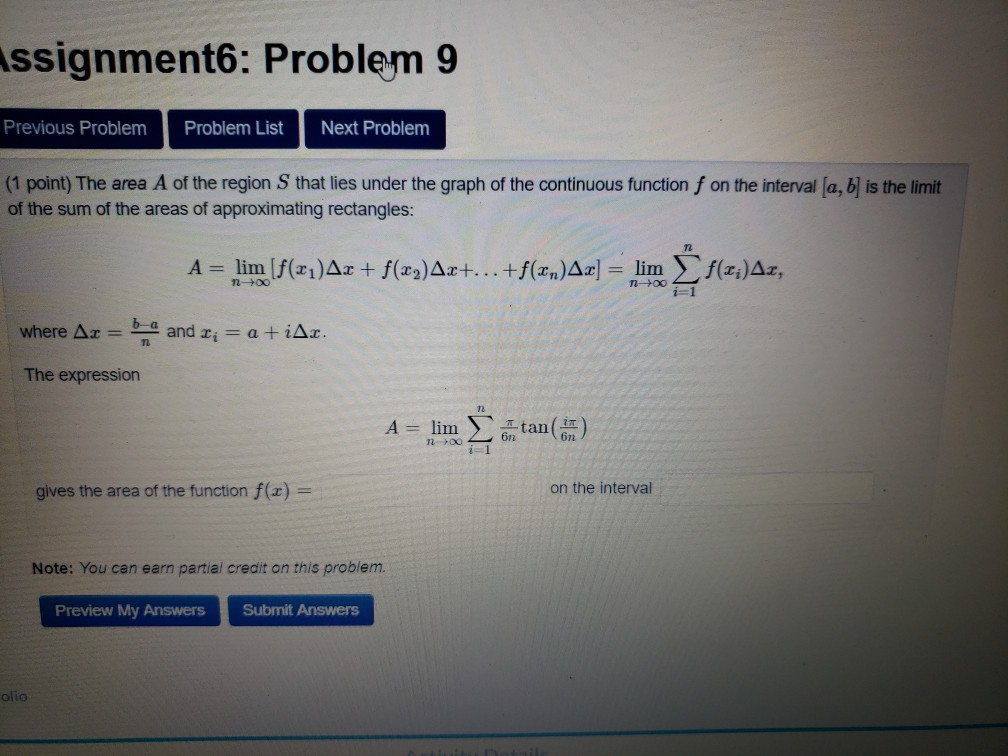ssignment6: Problem 9 Previous Problem Problem List Next Problem (1 point) The area A of the region Sthat lies under the graph of the continuous function f on the interval (a, b) is the limit of the sum of the areas of approximating rectangles: A = lim (f(21)Ar + f(x2)Ax+...+f(xn)Ax] = lim f(x;)Az, n-> ng i=1 where Ax = b and Ti = a +iAr. The expression A = lim Itan(n) 7200 6n2 gives the area of the function f(x) = on the interval Note: You can earn partial credit on this problem. Preview My Answers Submit Answers olio

We need at least 9 more requests to produce the answer.

1 / 10 have requested this problem solution

The more requests, the faster the answer.

All students who have requested the answer will be notified once they are available.

#### Earn Coins

Coins can be redeemed for fabulous gifts.

Similar Homework Help Questions
• ### Week 1: Problem 21 Previous Problem List Next (1 point) Definition: The AREA A of the...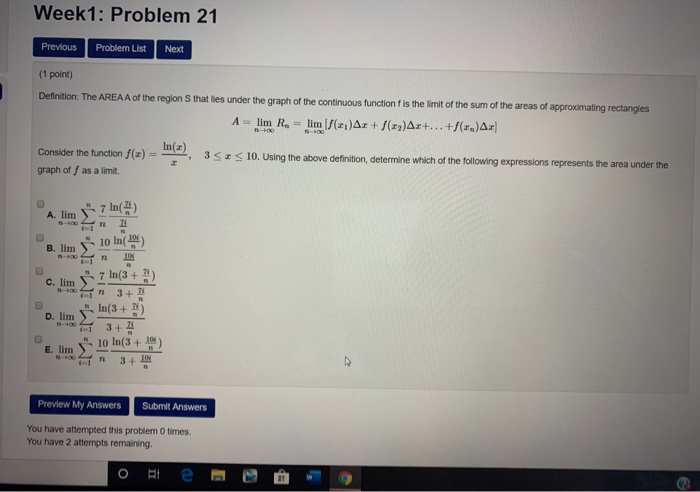Week 1: Problem 21 Previous Problem List Next (1 point) Definition: The AREA A of the region that lies under the graph of the continuous function is the limit of the sum of the areas of approximating rectangles A - lim R. - lim (/(x1)Ar + ()Ar+...+(2.)A: () = 352 10. Using the above definition determine which of the following expressions represents the area under the Consider the function graph off as a limit. A. lim j7 ln() lo in...

• ### (1 point) Definition: The AREA A of the region that lies under the graph of the...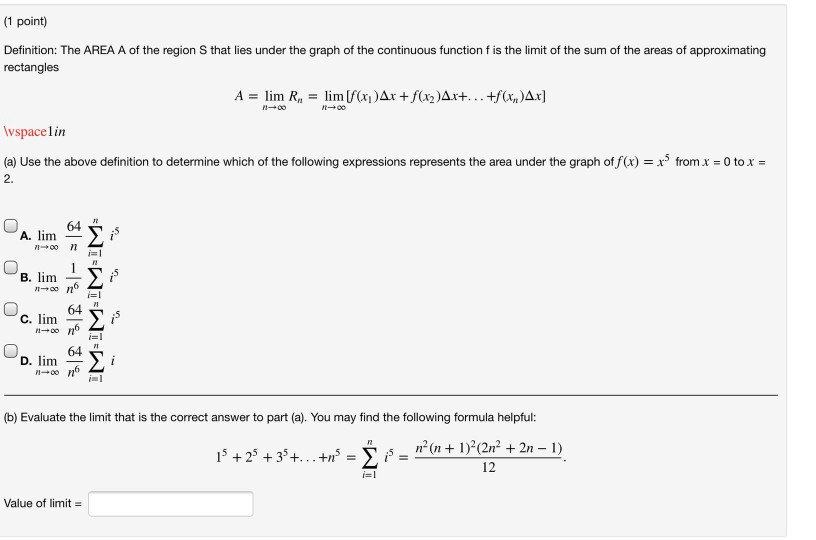(1 point) Definition: The AREA A of the region that lies under the graph of the continuous function f is the limit of the sum of the areas of approximating rectangles A = lim R, = lim [f(x)Ar + f(x2)Ax+... +f(x,y)Ax] 100 Wspacelin (a) Use the above definition to determine which of the following expressions represents the area under the graph of f(x) = x3 from x = 0 to x = 2. 64 A. lim 7100 11 i= B....

• ### send help for these 4 questions, please show steps Definition: The AREA A of the region...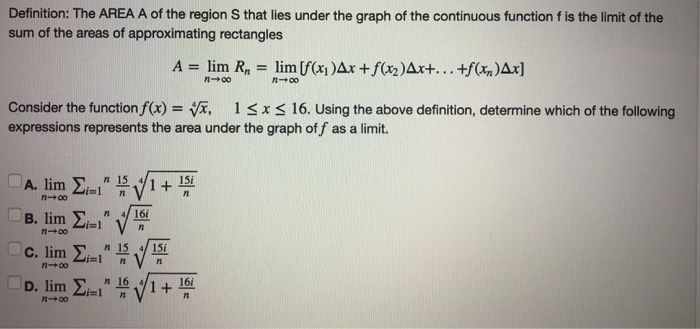send help for these 4 questions, please show steps Definition: The AREA A of the region S that lies under the graph of the continuous function f is the limit of the sum of the areas of approximating rectangles A = lim R, = lim [f(x)Ax +f(x2)Ax+...+f(x)Ax] - 00 Consider the function f(x) = x, 13x < 16. Using the above definition, determine which of the following expressions represents the area under the graph off as a limit. A. lim...

• ### -/2 POINTS SESSCALCET2 5.1.503.XP. The area A of the region that lies under the graph of...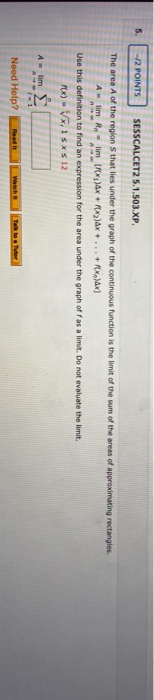-/2 POINTS SESSCALCET2 5.1.503.XP. The area A of the region that lies under the graph of the continuous function is the limit of the sum of the areas of approximating rectangles. lim Rn limf ax + 2)Ax + ... + X)x] Use this definition to find an expression for the area under the graph off as a limit. Do not evaluate the limit. FX) - VX,15* \$ 12 A lim Need Help? Talk to Tutor

• ### (1 point) In this problem you will calculate the area between f(x) = x2 and the...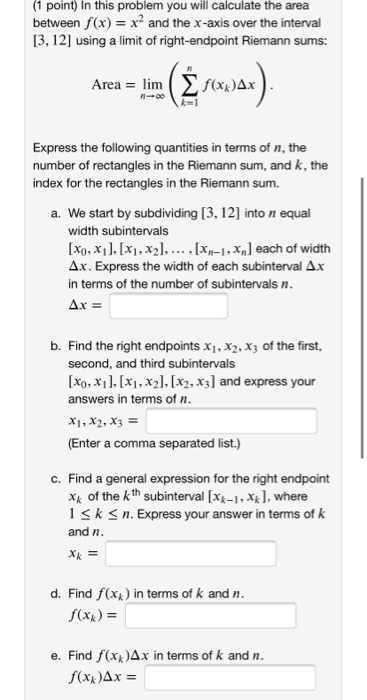(1 point) In this problem you will calculate the area between f(x) = x2 and the x-axis over the interval [3,12] using a limit of right-endpoint Riemann sums: Area = lim ( f(xxAx bir (3 forwar). Express the following quantities in terms of n, the number of rectangles in the Riemann sum, and k, the index for the rectangles in the Riemann sum. a. We start by subdividing [3, 12) into n equal width subintervals [x0, x1], [x1, x2),..., [Xn-1,...

• ### (2 points) The area A of the region S that lies under the graph of the...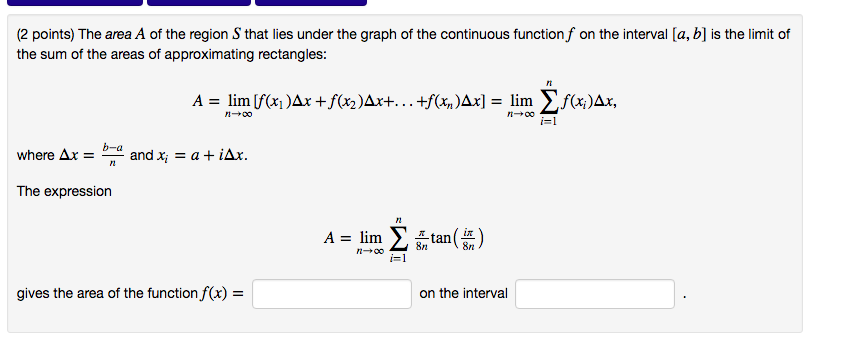(2 points) The area $$A$$ of the region $$S$$ that lies under the graph of the continuous function $$f$$ on the interval $$[a, b]$$ is the limit of the sum of the areas of approximating rectangles:$$A=\lim _{n \rightarrow \infty}\left[f\left(x_{1}\right) \Delta x+f\left(x_{2}\right) \Delta x+\ldots+f\left(x_{n}\right) \Delta x\right]=\lim _{n \rightarrow \infty} \sum_{i=1}^{n} f\left(x_{i}\right) \Delta x$$where $$\Delta x=\frac{b-a}{n}$$ and $$x_{i}=a+i \Delta x$$.The expression$$A=\lim _{n \rightarrow \infty} \sum_{i=1}^{n} \frac{\pi}{8 n} \tan \left(\frac{i \pi}{8 n}\right)$$gives the area of the function $$f(x)=$$ on...

• ### Assignment 8: Problem 9 Previous Problem List Next (1 point) Find the surface area of the...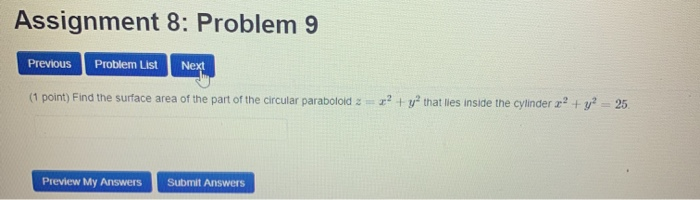Assignment 8: Problem 9 Previous Problem List Next (1 point) Find the surface area of the part of the circular paraboloid =? + y that lies inside the cylinder x? + y = 25. Preview My Answers Submit Answers

• ### HW-13: Problem 2 Previous Problem Problem List Next Problem (1 point) 2 2 Find the surface...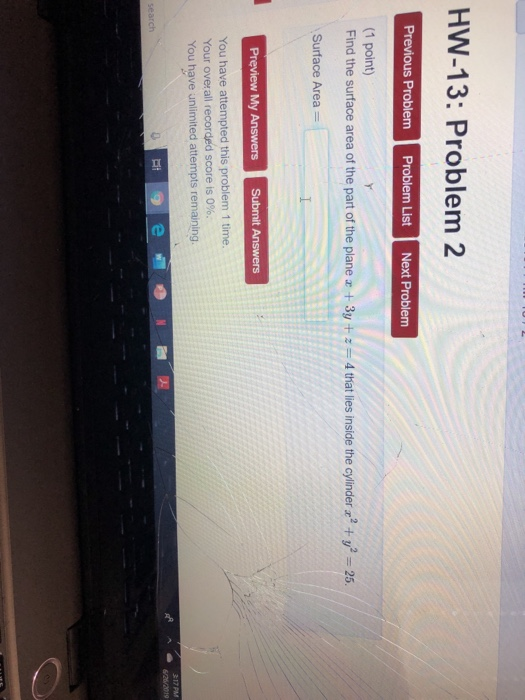HW-13: Problem 2 Previous Problem Problem List Next Problem (1 point) 2 2 Find the surface area of the part of the plane +3y + z=4 that lies inside the cylinder + y= 25. Surface Area Preview My Answers Submit Answers You have attempted this problem 1 time. Your overall recorded score is 0%. You have unlimited attempts remaining. 317 PM 6/26/2019 e search HW-13: Problem 2 Previous Problem Problem List Next Problem (1 point) 2 2 Find the surface...

• ### MA441-1.3-Limit-Laws: Problem 1 Previous Problem Problem List Next Problem (1 point) Using: lim f(x) = -3...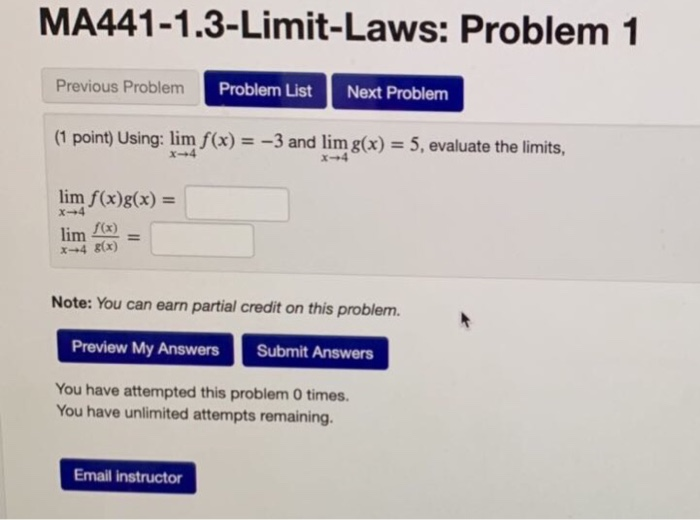MA441-1.3-Limit-Laws: Problem 1 Previous Problem Problem List Next Problem (1 point) Using: lim f(x) = -3 and lim g(x) = 5, evaluate the limits, lim f(x)g(x) = lim f(x) x +4 (x) Note: You can earn partial credit on this problem. Preview My Answers Submit Answers You have attempted this problem 0 times. You have unlimited attempts remaining. Email instructor

• ### Sec7.1: Problem 8 Previous Problem List Next (1 point) Book Problem 21 0 to 3 sin()...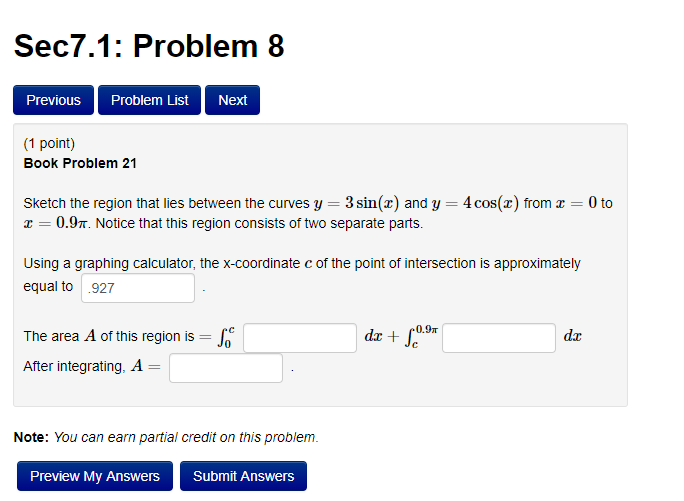Sec7.1: Problem 8 Previous Problem List Next (1 point) Book Problem 21 0 to 3 sin() and y = 4 cos(x) from Sketch the region that lies between the curves y 0.9. Notice that this region consists of two separate parts. Using a graphing calculator, the x-coordinate c of the point of intersection is approximately equal to 927 dx+ Se 0,9 dr The area A of this region is Jc After integrating, A = Note: You an earn partial credit...